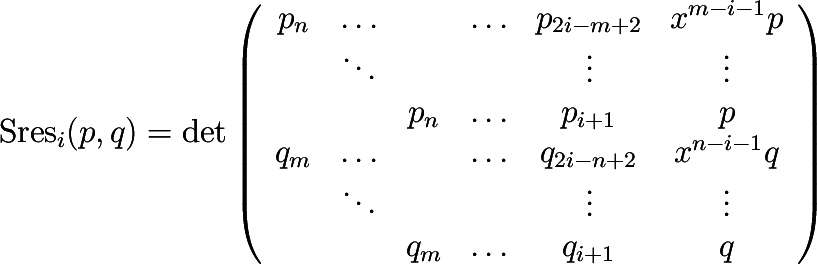CGAL 5.0.2 - Polynomial
PolynomialTraits_d::PolynomialSubresultants Concept Reference

## Definition

Computes the polynomial subresultant of two polynomials $$p$$ and $$q$$ of type PolynomialTraits_d::Polynomial_d with respect to outermost variable. Let $$p=\ccSum{i=0,\ldots,n}{} p_i x^i$$ and $$q=\ccSum{i=0,\ldots,m}{} q_i x^i$$, where $$x$$ is the outermost variable. The $$i$$-th subresultant (with $$i=0,\ldots,\min\{n,m\}$$) is defined by.where $$p_i$$ and $$q_i$$ are set to zero if $$i<0$$. In the case that $$n=m$$, $$\mathrm{Sres_n}$$ is set to $$q$$.

The result is written in an output range, starting with the $$0$$-th subresultant $$\mathrm{Sres}_0(p,q)$$ (aka as the resultant of $$p$$ and $$q$$).

Note
This functor is optional.
Refines:

AdaptableBinaryFunction

CopyConstructible

DefaultConstructible

Polynomial_d
PolynomialTraits_d
PolynomialTraits_d::Resultant
PolynomialTraits_d::PrincipalSubresultants
PolynomialTraits_d::PolynomialSubresultantsWithCofactors
PolynomialTraits_d::SturmHabichtSequence

## Operations

template<typename OutputIterator >
OutputIterator operator() (Polynomial_d p, Polynomial_d q, OutputIterator out)
computes the polynomial subresultants of $$p$$ and $$q$$, with respect to the outermost variable. More...

template<typename OutputIterator >
OutputIterator operator() (Polynomial_d p, Polynomial_d q, OutputIterator out, int i)
computes the polynomial subresultants of $$p$$ and $$q$$, with respect to the variable $$x_i$$.

## ◆ operator()()

template<typename OutputIterator >
 OutputIterator PolynomialTraits_d::PolynomialSubresultants::operator() ( Polynomial_d p, Polynomial_d q, OutputIterator out )

computes the polynomial subresultants of $$p$$ and $$q$$, with respect to the outermost variable.

Each element is of type PolynomialTraits_d::Polynomial_d.